# Atomic Structure

## Questions

#### Question 1(1984)

Define the terms : atomic number, mass number and electron.

Atomic number [Z] of an element is the number of :

• Protons - in the nucleus of an atom
• Electrons - in the complete atom
• Positive charges - in the nucleus of it's atom

Mass number [A] of an atom is the total number of protons and neutrons in the atom of an element.

Electron [e] is a subatomic particle revolving around the nucleus of atom having unit negative charge 1.602 x 10-19 coulombs, and mass of 9.107 x 10-28 g. It is 1⁄1837 of the mass of a hydrogen atom or proton.

#### Question 1(1985)

From the symbol $^4_2\text{He}$ for the element helium, write down the mass number and the atomic number of the element.

Mass number — 4

Atomic number — 2

#### Question 1(1986)

Sulphur has an atomic number of 16 and a mass number of 32. State the number of protons and neutrons present in the nucleus of sulphur.

Given,

Atomic number (Z) = 16 and

Atomic number = number of protons in the atom of an element.

Hence, number of protons = 16

Mass number (A) = 32 and

Mass number = number of protons and neutrons

Hence, number of protons and neutrons = 32

Number of Neutrons = A – Z = 32 – 16 = 16
Hence, Number of Neutrons = 16

#### Question 2(1986)

Give a simple diagram to show the arrangement of the electrons in an atom of sulphur.

Below diagram shows the arrangement of the electrons in an atom of sulphur: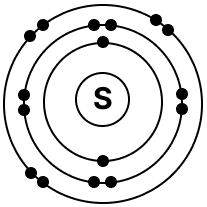#### Question 1(1987)

$^{24}_{12}\text{Mg}$ and $^{26}_{12}\text{Mg}$ are symbols of two isotopes of magnesium. Compare the atoms of these isotopes with respect to :

(i) the composition of their nuclei.

(ii) their electronic configurations.

(iii) give the reason why the two isotopes of magnesium have different mass numbers.

(i) The composition of their nuclei :

$^{24}_{12}\text{Mg}$ :

Atomic number [Z] = electron [e] = proton [p] = 12

Mass number [A] = 24

As, neutron [n] = A - Z = 24 - 12 = 12.

Hence, p = 12, n = 12

$^{26}_{12}\text{Mg}$ :

Atomic number [Z] = electron [e] = proton [p] = 12

Mass number [A] = 26

As, neutron [n] = A - Z = 26 - 12 = 14.

Hence, p = 12, n = 14

(ii) Their electronic configurations :

$^{24}_{12}\text{Mg}$ :

As electron [e] = 12 hence, electronic configuration = 2,8,2

$^{26}_{12}\text{Mg}$ :

As electron [e] = 12 hence, electronic configuration = 2,8,2

(iii) Reason for difference in mass numbers — As the number of neutrons is different in the two isotopes of magnesium, therefore, they have different mass numbers.

#### Question 2(1987)

Chlorine is an element of atomic number 17. It is a mixture of two isotopes having mass number of 35 and 37.

(i) What is meant by 'atomic number of an element' ? What do you understand by an 'atom'.

(ii) Write down the electronic configuration of the chlorine atom.

(iii) State the number of protons, electrons and neutrons in the following isotopes: $^{35}\text{Cl}_{17}$, $^{37}\text{Cl}_{17}$

(iv) Explain why the two atoms in (iii) above have the same chemical reactions.

(v) If molten magnesium chloride is electrolyzed suggest a suitable electrode [anode].

(i) Atomic number [Z] of an element is the number of :

• Protons - in the nucleus of an atom
• Electrons - in the complete atom
• Positive charges - in the nucleus of it's atom

An atom is the smallest particle of an element which can take part in a chemical reaction and is considered the basic unit of matter. e.g. O,Cl are atoms.

(ii) Atomic number of Chlorine is 17 so it has 17 electrons. Hence, its electronic configuration = 2, 8, 7

(iii) $^{35}\text{Cl}_{17}$

• No. of protons = 17
• No. of electrons = 17
• No. of neutrons = A - Z = 35 - 17 = 18

$^{37}\text{Cl}_{17}$

• No. of protons = 17
• No. of electrons = 17
• No. of neutrons = A - Z = 37 - 17 = 20

(iv) As the two atoms in (iii) above are isotopes and hence have same electronic configuration. Therefore, they have similar chemical properties because chemical properties are dependent on electronic configuration of an element.

(iv) Carbon anode is used when molten magnesium chloride is electrolyzed.

#### Question 1(1988)

Five atoms are labelled V to Z

AtomsMass NumberAtomic number
V4020
W199
X73
Y168
Z147

(i) Which one of these atoms (1) contains 7 protons; (2) has an electronic configuration 2, 7 ?

(ii) Write down the formula of the compound formed by atoms X and Y.

(i) As Z has atomic number of 7, hence it has 7 protons. Electronic configuration of 2, 7 means the atom has 2 + 7 = 9 electrons. Therefore, element W has electronic configuration 2, 7 since its atomic number is 9.

(ii) Number of electron in X is 3. Therefore, electronic configuration = (2, 1). As X will try to lose 1 electron to attain stable state, hence, X has valency [1+]

Number of electron in Y is 8. Therefore, electronic configuration = (2, 6). As Y will try to gain 2 electrons to attain stable state, hence, Y has valency [2-]

Since valency of X is 1+ and valency of Y is 2-

Formula of the compound:

$\text{X}^{1+} \phantom{\nearrow} \text{Y}^{2-} \\[0.5em] \overset{\phantom{1}{1}}{\text{X}} \space {\searrow}\mathllap{\swarrow} \space \overset{2}{\text{Y}} \Rightarrow \underset{\phantom{2}{2}}{\text{X}} \space {\searrow}\mathllap{\swarrow} \space \underset{1}{\text{Y}} \\[0.5em]$

So, we get the formula as $\bold{X}_2\bold{Y}$

#### Question 1(1989)

Elements X, Y, Z have atomic numbers 6, 9 and 12 respectively. Which one :

(i) forms anion – negative ion;

(ii) forms cation – positive ion;

(iii) has 4 electrons in the outermost orbit.

X = Electronic configuration [2,4]
Y = Electronic configuration [2,7]
Z = Electronic configuration [2,8,2]

(i) Y gains one electron to attain a stable octet, hence becomes negatively charged anion.

(ii) Z loses two electrons to attain a stable octet hence becomes positively charged cation.

(iii) X has 4 electrons in the outer most shell.

#### Question 2(1989)

Ordinary chlorine gas has two isotopes : $^{35}_{17}\text{Cl}$ and $^{37}_{17}\text{Cl}$ in the ratio of 3 : 1. Calculate the relative atomic mass [atomic weight] of chlorine.

Given, $^{35}_{17}\text{Cl}$ and $^{37}_{17}\text{Cl}$ are in the ratio of 3 : 1

Therefore, ratio of atomic masses = 3:1

Total mass = [3 x 35] + [1 x 37] = 105 + 37 = 142

Relative atomic mass of chlorine = $\dfrac{\text{Total mass}}{3 + 1}$

= $\dfrac{142}{4}$ = 35.5

#### Question 1(1990)

The atom of aluminium is represented by 27Al13. Write down the number of :

(a) electrons

(b) protons

(c) neutrons

(d) the arrangement of electrons

in the different orbits or shells in one atom of aluminium.

27Al13

Z = 13 , A = 27

(a) electrons = Z = 13

(b) protons = Z = 13

(c) neutrons = A - Z = 27 - 13 = 14

(d) the arrangement of electrons = K, L, M

#### Question 2(1990)

Name the element which does not contain any neutrons in it's nucleus.

Hydrogen 1H1

#### Question 3(1990)

Elements A, B and C have atomic numbers 9, 20 and 10 respectively.

(i) State which one is (1) a non-metal; (2) a metal; (3) chemically inert.

(ii) Write down the formula of the compound formed by two of the above elements.

(i) The electronic configurations of the elements are:
A = [2, 7]
B = [2, 8, 8, 2]
C = [2, 8]

(1) A is a non-metal as it will gain electron to attain a stable octet.

(2) B is a metal as it will lose two electrons to attain a stable octet.

(3) C is chemically inert as it's valence shell is completely filled.

(ii) A has 7 electrons in the outermost shell. Therefore, it will try to gain one electron to attain stable octet, hence, A has valency [1-]

As B has 2 in the outermost shell. Therefore, it will try to lose 2 electrons to attain stable octet, hence, B has valency [2+]

Since valency of A is 1- and valency of B is 2+

Formula of the compound:

$\text{B}^{2+} \phantom{\nearrow} \text{A}^{1-} \\[0.5em] \overset{\phantom{2}{2}}{\text{B}} \space {\searrow}\mathllap{\swarrow} \space \overset{1}{\text{A}} \Rightarrow \underset{\phantom{1}{1}}{\text{B}} \space {\searrow}\mathllap{\swarrow} \space \underset{2}{\text{A}} \\[0.5em]$

So, we get the formula as $\bold{BA}_\bold{2}$

#### Question 4(1990)

Define : Proton, Electron, Neutron.

Proton [p] is a subatomic particle in the nucleus of an atom having unit positive charge 1.602 x 10-19 coulombs and mass of 1.670 x 10-24 g. It is 1837 times as heavy as an electron.

Electron [e] is a subatomic particle revolving around the nucleus of atom having unit negative charge 1.602 x 10-19 coulombs, and mass of 9.107 x 10-28 g. It is 1⁄1837 of the mass of a hydrogen atom or proton.

Neutrons [n] is a subatomic particle found in the nucleus of an atom, carrying no electric charge and mass equal to the mass of proton i.e., 1.676 x 10-24 g.

#### Question 1(1991)

Write down the electronic configuration of the following:

(i) $^{27}_{13}\text{X}$,

(ii) $^{35}_{17}\text{Y}$.

Write down the number of electrons in X and neutrons in Y and the formula of the compound formed by X and Y.

(i) $^{27}_{13}\text{X}$ = [2, 8, 3] ; Number of electrons in X = 13

(ii) $^{35}_{17}\text{Y}$ = [2, 8, 7] ; Number of neutrons = A - Z = 35 - 17 = 18

Valency of $^{27}_{13}\text{X}$ = [2, 8, 3] = 3 positive

Valency of $^{35}_{17}\text{Y}$ = [2, 8, 7] = 1 negative.

Formula of the compound:

$\text{X}^{3+} \phantom{\nearrow} \text{Y}^{1-} \\[0.5em] \overset{\phantom{3}{3}}{\text{X}} \space {\searrow}\mathllap{\swarrow} \space \overset{1}{\text{Y}} \Rightarrow \underset{\phantom{1}{1}}{\text{X}} \space {\searrow}\mathllap{\swarrow} \space \underset{3}{\text{Y}} \\[0.5em]$

So, we get the formula as $\bold{XY}_\bold{3}$

#### Question 1(1992)

According to the Dalton's Atomic Theory, atoms of the same element are identical in all respects. But according to the Modern Atomic Theory, this postulate is proved wrong. Explain.

According to modern atomic theory atoms of the same element may not be alike in all respect as seen in case of isotopes which are atoms of the same element having same atomic number but different mass numbers.

e.g. isotopes of chlorine $^{35}_{17}\text{Cl}$ and $^{37}_{17}\text{Cl}$ have different atomic masses 35 and 37. This proves Dalton's Atomic Theory wrong.

#### Question 1(1993)

What are isotopes.

Isotopes are atoms of the same element having same atomic number but different mass number.

#### Question 2(1993)

Write down :

(i) the mass number of the atom having 20 neutrons and 15 protons.

(ii) the number of neutrons in the nucleus of an atom having atomic number 17 and mass number 37.

(i) Mass number (A) = Neutrons (n) + Protons (p) = 20 + 15 = 35

(ii) Number of neutrons = n = A -Z = 37 – 17 = 20

#### Question 1(1994)

What is a proton ? What is the significance of the number of protons found in the atoms of each of the different elements.

Proton [p] is a subatomic particle in the nucleus of an atom having unit positive charge 1.602 x 10-19 coulombs and mass of 1.670 x 10-24 g. It is 1837 times as heavy as an electron.

As elements are arranged on the basis of the number of protons in their respective atoms, therefore, proton helps in determining and understanding the structure and behaviour of an atom. Protons also determine the positive charge of the nucleus in the atom.

#### Question 2(1994)

What is the relation between the number of protons and the number of electrons in an atom.

The number of protons in an atom is equal to the number of electrons.

#### Question 3(1994)

What would be the reason for an element to have atoms with differing mass numbers.

Atoms of same element have different mass number due to the difference in number of neutrons.

#### Question 4(1994)

Copy and complete the following table relating to the atomic structure of some elements :

ElementAtomic No.Mass No.No. of ProtonsNo. of NeutronsNo. of Electrons
Beryllium49
Fluorine9    10
Sodium      1211
Aluminium  27    13
Phosphorus  3115

ElementAtomic No.Mass No.No. of ProtonsNo. of NeutronsNo. of Electrons
Beryllium49454
Fluorine9199109
Sodium1123111211
Aluminium1327131413
Phosphorus1531151615

#### Question 5(1994)

The electronic structure [configuration] of fluorine can be written as 2, 7. In a similar way give the electronic configuration of :

(i) aluminium

(ii) phosphorus

Electronic configuration of :

(i) Aluminium has atomic number = 13. Therefore, electronic configuration is 2, 8, 3.

(ii) Phosphorus has atomic number = 15. Therefore, electronic configuration is 2, 8, 5

#### Question 1

State the main postulates of Dalton's atomic theory. How does the modern atomic theory contradict and correlate with Dalton's atomic theory.

Postulates of Dalton's Atomic Theory:

• Matter consist of small indivisible particles called atoms.
• Atoms are neither created nor destroyed.
• Atoms of the same element are alike in every aspect and differ from atoms of all other elements.
• Atoms combine with other atoms in simple whole number ratio's forming compound atoms or molecules.
• An atom is the smallest unit of matter which takes part in a chemical reaction and all chemical changes result from separation or combination of atoms.

Contradictions with Modern atomic theory:

Dalton's atomic theoryModern atomic theory
Atoms are indivisible and indestructible.Atoms are divisible and destructible.
An atom is the smallest indivisible particle of an elementAtoms are divisible - consisting of subatomic particles like electrons, protons and neutrons.
Atoms of the same element are alike in all respects and differ from atoms of other elements.Atoms of the same element may not be alike in all respects as seen in the case of isotopes which are atoms of the same element having same atomic number but different mass numbers.

Correlation with Modern atomic theory:

The modern atomic theory however correlates with Dalton's atomic theory in the fact that atoms are the smallest unit of matter taking part in a chemical reaction and in a given compound the relative number and kind of atoms is a constant.

#### Question 2

Explain in brief the experimental proofs which led to the discovery of –

(i) Electrons

(ii) Protons

(iii) atomic nucleus

(iv) neutrons.

(i) Electrons — Experimental proofs leading to the discovery of Electrons were given by William Crookes in 1878 and Sir J.J. Thomson in 1897.

• William Crookes found that when an electric discharge is passed through a tube containing a gas at low pressure (0.01 mm of Hg), blue rays were emitted from the cathode and were termed cathode rays.
• Sir J.J. Thomson studied the characteristics and constituents of cathode rays and found that:
• Cathode rays travel in straight lines from cathode to anode and cast a shadow of the object placed in their path.
• They are deflected by magnetic and electric field [attracted towards positively charged plate].
• They have kinetic energy and raise the temperature of a metallic object on which they fall.

These findings lead to the conclusion that Cathode rays consist of negatively charged particles now called electrons.

(ii) Protons : Goldstein discovered protons.

• As atoms are electrically neutral there must be equal positively charged particles in atom. This led to discovery of the protons.
• He used a perforated disc as cathode and found positively charged rays travelling in opposite direction to cathode rays.
• These rays consisted of positively charged particles which led to the discovery of protons.

(iii) Atomic Nucleus : Lord Rutherford discovered atomic nucleus in 1911.

• He directed alpha particles towards a metallic sheet.
• The deflection of the alpha particles was observed and concluded that there is a nucleus in the centre of the atom.

(iv) Neutrons : James Chadwick discovered neutron in 1932.

• He established that an atom contains protons and electrons.
• Electrons have negligible mass, hence the mass is concentrated in the nucleus. ∴ Atomic mass = mass of protons.
• However, in the case of helium - there are two protons in it's nucleus and hence atomic mass should be equal to twice the mass of each proton i.e., 2 x 1.008 but it's atomic mass is calculated experimentally and found to be 4.003 a.m.u.
• It was therefore proved that, in the nucleus of an atom, there is other particle called a neutron which has no electrical charge but is almost of an equal mass as the protons.
• This confirmed the existence of neutrons

#### Question 3

State in brief the drawbacks of Rutherford's atomic model correlating them with the postulates of Bohr's atomic model.

Drawbacks of Rutherford's Atomic Model :

• In Rutherford's model of the atom - the electrons revolve around the nucleus and the attractive force of the positively charged nucleus would be balanced by the centrifugal force arising due to the rotation of electron.
• If an electron moves round the nucleus - it must radiate out energy and gradually move towards the nucleus following a spiral path - till it collides and ultimately collapses in it.
• Thus, Rutherford's model could not explain correctly the stability of an atom.

Postulates of Bohr's Atomic Model :

• Electrons revolve around the nucleus in fixed orbits or energy levels, possessing certain amount of energy.
• The integer n represents the various energy levels 1, 2, 3 or K, L, M starting from the innermost.
• As long as the electrons rotates in one of the energy levels - it neither loses or absorbs energy. When energy is supplied initially to the atoms - an electron moves to an orbit of higher energy. When this electron drops back to the original orbit - energy is radiated by the atom - according to equation E2 - E1 = hγ

[E2 and E1 are energy states of the electron in higher and lower orbit.]

Bohr's model gave the preciseness of location and mode of rotation of electron around the nucleus.

#### Question 4

What is meant by the terms :

(i) subatomic particles,

(ii) nucleus,

(iii) orbits,

(iv) atomic number

(v) mass number with reference to an atom.

(i) Subatomic particles : The particles into which an atom is divided (i.e., protons, electrons, neutrons) are called the subatomic particles.

(ii) Nucleus : The centre or the nucleus of the atom is the place where the protons and neutrons are found.

(iii) Orbits : The electrons revolve around the nucleus along imaginary paths known as shells or more accurately orbits or orbitals.

(iv) Atomic number : Number of protons or number of electrons present in an atom. [Z]

(v) Mass number [A] : The Mass number of an atom is the total number of protons and neutrons collectively called nucleons in the nucleus of the atom.

#### Question 5

Represent each of the following :

(i) a proton 'p'

(ii) an electron 'e'

(iii) a neutron 'n'

in terms of it's symbols showing the subscript and superscript values.

(i) Proton : $^{\enspace 1}_{+1}\text{p}$

(ii) Electron : $^{\enspace 0}_{-1}\text{e}$

(iii) Neutron : $^1_0\text{n}$

#### Question 6

What are 'energy levels'? Explain the arrangement and distribution of electrons in the various shells with reference to an atom in general and to an atom of potassium $^{39}_{19}\text{K}$ with special reference to the 2n2 rule.

Electrons revolve around the nucleus in fixed orbits. Each orbit is associated with a certain amount of energy hence named 'energy levels'.

Arrangement of electrons :

• The shell closest to the nucleus is called K shell [first shell or number one shell].
• The next shell is called the L shell [second shell or number 2 shell]
• Following shells are M,N...etc.
• An electron has minimum energy in the first or K shell and the energy increase in the order K, L, M, N.
• The maximum number of electrons which can be present in any shell of an atom is given by or represented by a formula 2n2, where n is equal to the number of the shell as counted from the nucleus.
ShellShell
No.[n]
Formula
2n2
Max
Electrons
K12 × (1)22
L22 × (2)28
M32 × (3)218
N42 x (4)232
• The outer most shell cannot have more than 8 electrons.
• The penultimate shell [last but one ] cannot have more than 18 electrons.
• A new shell is formed as soon as the - outermost shell attains 8 electrons.

With reference to Potassium $^{39}_{19}\bold{K}$ :

No. of Electrons [e] = 19
No. of Protons [p] = 19
No. of Neutrons [n] = A - Z
= 39 - 19 = 20

The two rules governing the distribution of electrons are:

1. Rule 1 — Maximum number of electrons in each shell is given by the formula 2n2
2. Rule 2 — The outermost shell cannot have more than 8 electrons.

As per rule 1 (2n2 rule), distribution of electrons for Potassium will be:

ShellElectrons
K2
L8
M9
[Last Shell]

But as per rule 2, outermost shell cannot have more than 8 electrons. So M shell will have 8 electrons and a new shell (N) will be formed with 1 electron. Hence, the final electronic configuration of Potassium will be:

ShellElectrons
K2
L8
M8
N1

∴ Electronic configuration of Potassium = 2, 8, 8, 1

#### Question 7

An element 'A' has mass number 23 and atomic number 11. State the —

(i) no. of neutrons in it's shell,
(ii) electronic configuration of the element 'A'.

Given, mass number 23 and atomic number 11. So, Protons (P) = 11 and

(i) Neutrons (n) = A - P = 23 - 11 = 12

(ii) As number of electrons (e) = 11

∴ Electronic configuration of A = 2, 8, 1

#### Question 8

The following elements U to Z are given 3U, 6V, 9W, 14X, 18Y, 20Z

State the electronic configuration of each and state whether they are metals, non-metals or inert gases.

ElementElectronic configurationmetals, non-metals or inert gases
3U2,1Metal
6V2,4Non-metal [s]
9W2,7Non-metal [g]
14X2,8,4Metalloid
18Y2,8,8Inert gas
20Z2,8,8,2Metal

#### Question 9

Draw the geometric atomic structure of each of the following atoms showing the number of electrons, protons and neutrons in each of them :

(i) $^{12}_6 \text{C}$

(ii) $^{23}_{11} \text{Na}$

(iii) $^{31}_{15}\text{P}$

(iv) $^{39}_{19} \text{K}$

(v) $^{40}_{20} \text{Ca}$

(i) Atom of Carbon [$^{12}_6 \text{C}$] —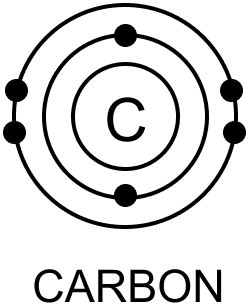No. of Electrons [e] = 6
No. of Protons [p] = 6
No. of Neutrons [n] = A - Z
= 12 - 6 = 6
Electronic configuration = 2, 4

(ii) Atom of sodium $^{23}_{11} \text{Na}$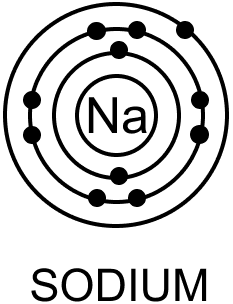No. of Electrons [e] = 11
No. of Protons [p] = 11
No. of Neutrons [n] = A - Z
= 23 - 11 = 12
Electronic configuration = 2, 8, 1

(iii) Atom of Phosphorus $^{31}_{15}\text{P}$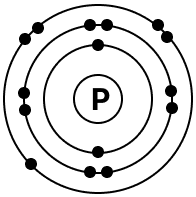No. of Electrons [e] = 15
No. of Protons [p] = 15
No. of Neutrons [n] = A - Z
= 31 - 15 = 16
Electronic configuration = 2, 8, 5

(iv) Atom of Potassium $^{39}_{19} \text{K}$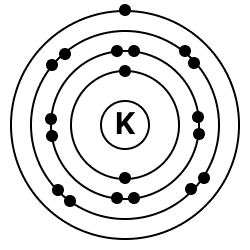No. of Electrons [e] = 19
No. of Protons [p] = 19
No. of Neutrons [n] = A - Z
= 39 - 19 = 20
Electronic configuration = 2, 8, 8, 1

(v) Atom of Calcium $^{40}_{20} \text{Ca}$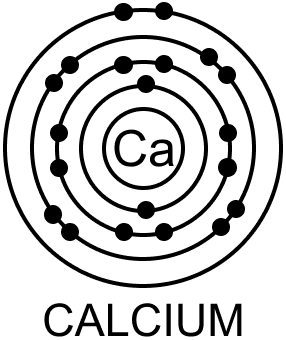No. of Electrons [e] = 20
No. of Protons [p] = 20
No. of Neutrons [n] = A - Z
= 40 - 20 = 20
Electronic configuration = 2, 8, 8, 2

#### Question 10

Define an 'isotope'. Give reasons why isotopes have same chemical but different physical properties.

Isotopes are atoms of the same element having same atomic number but different mass numbers.

Chemical properties of isotopes of the same element are similar because chemical properties are dependent on the electronic configuration of an atom. As Isotopes have same atomic number so they have same number of electrons and hence same electronic configuration.

Physical properties of isotopes of the same element are different because physical properties are dependent on the atomic mass. Isotopes have different mass number [A], different number of neutrons and hence different atomic masses giving them different physical properties.

#### Question 11

Draw the geometric atomic structure of the three isotopes of hydrogen and the two isotopes of chlorine.

Geometric atomic structures of three isotopes of hydrogen is shown in the diagram below: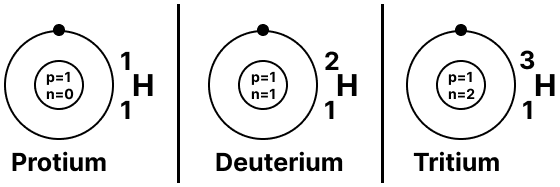Geometric atomic structures of two isotopes of chlorine is shown in the diagram below: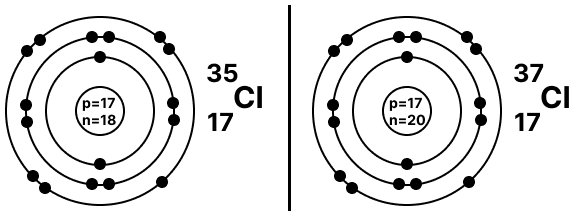#### Question 12

Four elements A, B, C, D are given :

A shows the presence of 20 neutrons, 17 protons and 17 electrons.

B shows the presence of 18 neutrons, 17 protons and 17 electrons.

C shows the presence of 10 neutrons, 9 protons and 10 electrons.

D shows the presence of 4 neutrons, 3 protons and 2 electrons.

State which of the above is —

(a) an anion

(b) a cation

(c) a pair of isotopes.

Write the formula of the compound formed between D and C.

(a) C is an anion.
Reason — C has 10 electrons but 9 protons. It means that C accepted 1 electron to achieve a stable electronic configuration. Hence, it is an anion.

(b) D is a Cation.
Reason — D has 2 electrons but 3 protons. It means that D donated one of its electrons to achieve a stable electronic configuration. Hence, it is a cation.

(c) A and B form a pair of isotopes.
Reason — Both A and B have the same number of protons and electrons i.e., 17 but differ in the number of neutrons. Hence, they form a pair of isotopes.

Formula of the compound formed between D and C:

D donates one electron and C accepts one electron. Hence, formula of compound formed between D and C is DC.

#### Question 13

What are noble gases. Give a reason why noble gases have stable electronic configuration.

Noble gases have stable electronic configuration i.e., their valence shell is complete. They do not gain, lose or share electrons. Their atoms are extremely unreactive.

For an atom to achieve stable electronic configuration, it must have:

1. Two electrons in the first shell (outermost) like that of noble gas Helium. This is termed the Duplet Rule.
2. Eight electrons in the outermost shell like that of noble gases other than Helium. This is termed the Octet Rule.

The octet and duplet arrangement of elements represents stability and hence noble gases have stable electronic configuration.

#### Question 14

Explain the reason for chemical activity of an atom with reference to it's electronic configuration.

Reasons for chemical activity of an atom are:

1. Unstable electronic configuration — Atoms of elements other than noble gases are assumed to have unstable electronic configuration.
2. Attaining stable electronic configuration — The driving force in combination of atoms is related to the tendency for them to attain a stable electronic configuration of the nearest noble gas.

#### Question 15.1

Differentiate between the terms —

Stable and unstable electronic configuration

Stable Electronic ConfigurationUnstable Electronic Configuration
Atoms having their valence shell completely filled are said to have Stable Electronic Configuration.Atoms which do not have their valence shells completely filled are said to have Unstable Electronic Configuration.
Atoms of noble gases have Stable Electronic Configuration.Atoms of elements other than noble gases have Unstable Electronic Configuration.
In atoms with Stable Electronic Configuration, electrons are arranged as per Duplet or Octet rule.In atoms with Unstable Electronic Configuration, arrangement of electrons does not follow Duplet or Octet rule.

#### Question 15.2

Differentiate between the terms —

Duplet and octet rule.

Duplet RuleOctet Rule
For an atom to achieve stable electronic configuration it must have 2 electrons in the first shell [outermost] like that of noble gas Helium.For an atom to achieve stable electronic configuration it must have 8 electrons in outermost orbit like that of noble gases other than Helium.

#### Question 16

Explain the octet rule for formation of —

(a) Sodium chloride from a sodium atom and a chlorine atom.

(b) Nitrogen molecule from two nitrogen atoms.

(a) As Na atom has atomic number 11 and electronic configuration [2, 8, 1]. Therefore, it tends to lose 1 electron from its valence shell forming Na1+ (cation) to attain a stable octet configuration of the nearest noble gas Neon [2, 8].

Cl, on the other hand has atomic number 17 and electronic configuration [2, 8, 7]. Therefore, it tries to gain one electron in its valence shell forming Cl1- (anion) to attain a stable octet configuration of the nearest noble gas Argon [2, 8, 8].

The oppositely charged cation and anion attract each other with a force - [electrostatic force of attraction] resulting in formation of an ionic or electrovalent compound [NaCl] as shown below.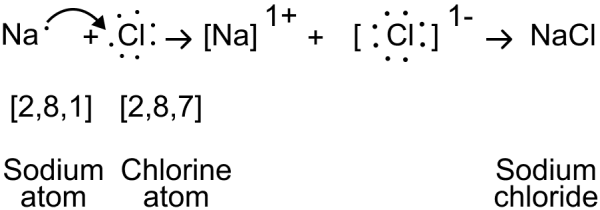(b) Nitrogen atom (147N) has atomic number 7 and electronic configuration [2, 5]. To attain stable octet structure of the nearest noble gas Neon [2, 8], Nitrogen needs three electrons in the valence shell. Nitrogen atom shares electron pairs with other nitrogen atom resulting in the formation of a molecular or covalent bond as shown below: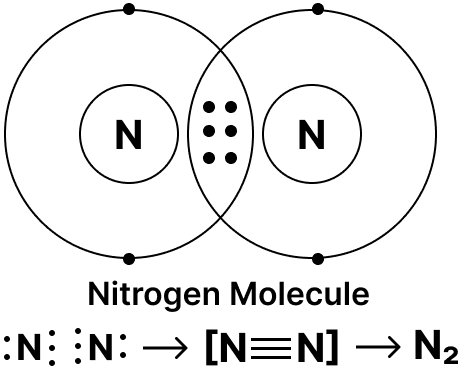## Unit Test Paper 4 — Atomic Structure

#### Question 1

Select the correct answer from the answers in brackets to complete each sentence.

1. An element has electronic configuration 2, 8, 1 and 12 neutrons. Its mass no. is ............... [11/23/12]
2. The maximum number of electrons in M-shell is ............... [8/32/18]
3. Isotopes have same ............... [no. of neutrons / electronic configuration / atomic masses].
4. An ............... [atom/ion] is capable of independent existence in solution.
5. An atom with electronic configuration 2, 7 and mass number 19 will have ............... neutrons. [8/10/12]

1. An element has electronic configuration 2, 8, 1 and 12 neutrons. It's mass no. is 23.
2. The maximum number of electrons in M-shell is 18.
3. Isotopes have same electronic configuration.
4. An ion is capable of independent existence in solution.
5. An atom with electronic configuration 2, 7 and mass number 19 will have 10 neutrons.

#### Question 2.1

Give reasons for the following.

The physical properties of isotopes of the same element are not identical.

Physical properties of isotopes of the same element are different because physical properties are dependent on the atomic mass. Isotopes have different mass number [A], different number of neutrons and hence different atomic masses giving them different physical properties.

#### Question 2.2

Give reasons for the following.

The mass number of an atom is slightly less than the actual atomic mass.

The mass number is a whole number approximation of the atomic mass in atomic mass units since the mass of the proton as well as the mass of the neutrons is approximately equal to 1 a.m.u. and the mass of the electron is negligible. Hence, the mass number is slightly less than the actual atomic mass.

#### Question 2.3

Give reasons for the following.

The shells surrounding the nucleus of an atom are also called 'energy levels'.

Each shell is associated with a certain amount of energy. As long as the electron rotates in one of the energy levels, it neither loses nor absorbs energy. On gaining energy electron moves to a higher energy shell and on losing energy it drops back to the original energy shell radiating the extra energy. Hence, the shells surrounding the nucleus of an atom are also called 'energy levels'.

#### Question 2.4

Give reasons for the following.

Helium is chemically extremely unreactive.

Helium has 2 electrons in the outermost shell [K shell], and duplet arrangement represents stability hence, helium is extremely unreactive.

#### Question 2.5

Give reasons for the following.

Mass number is slightly less than the actual atomic mass.

The mass number is a whole number approximation of the atomic mass in atomic mass units since the mass of the proton as well as the mass of the neutron is approximately equal to 1 a.m.u. and the mass of the electron is negligible. Hence, the mass number is slightly less than the actual atomic mass.

#### Question 3.1

Differentiate between the following terms.

Electron and proton

ElectronProton
Electron [e] is a subatomic particle revolving around the nucleus of the atom in a fixed orbit.Proton [p] is a subatomic particle present inside the nucleus of an atom.
Electron has a unit negative charge of 1.602 x 10-19 coulombs.Proton has a unit positive charge of 1.602 x 10-19 coulombs
Mass of electron is 9.107 x 10-28 g. It is 1⁄1837 of the mass of a hydrogen atom or a proton.Mass of proton is 1.670 x 10-24 g. It is 1837 times as heavy as an electron.

#### Question 3.2

Differentiate between the following terms.

Atomic number and mass number

Atomic numberMass number
Atomic number [Z] is equal to the number of protons in the atom of an element
OR
It is also equal to the number of electrons in the atom of an element.
Mass number [A] of an atom is the total number of protons and neutrons in the nucleus of an atom.

#### Question 3.3

Differentiate between the following terms.

Nucleus and nucleons

NucleusNucleons
The protons and neutrons are concentrated together at the centre of the atom called the Nucleus which is associated with all the positive charge of the atom.Protons and neutrons are together known as Nucleons.

#### Question 3.4

Differentiate between the following terms.

Valence shell and penultimate shell

Valence shellPenultimate shell
It is the outermost shell of an atom.It is the last but one shell of an atom.

#### Question 3.5

Differentiate between the following terms.

Octet and duplet

Octet RuleDuplet Rule
For an atom to achieve stable electronic configuration it must have 8 electrons in outermost orbit like that of noble gases other than Helium.For an atom to achieve stable electronic configuration it must have 2 electrons in the first shell [outermost] like that of noble gas Helium.

#### Question 4

Name or state the following.

1. The three isotopes of hydrogen.
2. Two elements having same number of protons and electrons but different number of neutrons.
3. The valency of an element whose electronic configuration is 2, 8, 3.
4. The shell closest to the nucleus of an atom.
5. An element having valency zero.

1. Protium [$^1_1\text{H}$], Deuterium [$^2_1\text{H}$], Tritium [$^3_1\text{H}$]
2. Sodium [e = p = 11, n = 12] and Chlorine [e = p = 17, n = 18]
3. The valency is 3 positive. Electronic configuration = 2, 8, 3. Therefore, the element tends to lose 3 electrons and gain stable octet configuration.
4. K shell.
5. Noble gases [He, Ne, Ar etc.]

#### Question 5

State the number of neutrons in each of the atoms A to E. Also state which of the atoms A to E is a metal.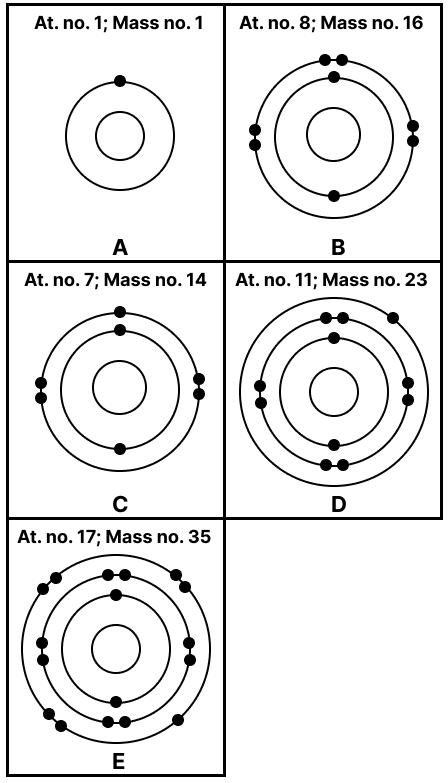Number of neutrons [n] = Mass number [A] – Atomic number [Z]

A → n = 1 – 1 = 0

B → n = 16 – 8 = 8

C → n = 14 – 7 = 7

D → n = 23 – 11 = 12

E → n = 35 – 17 = 18

D is a metal as it has the electronic configuration [2, 8, 1] and will give one electron to attain a stable octet.

#### Question 6

Match the elements A to E in List 1 with their valencies in List 2 and with their nature in List 3.

List 1
[Elements]
List 2
[Valency]
List 3
A: At. no. 7, Mass no.141. -3X: Metal
B: Elec. config. 2,82. +1Y: Non-metal
C: Neutrons 14, electrons 133. +3Z: Noble gas
D: Neutrons 22, protons 184. +2
E: Elec. config. 2,8,15. 0